# Adapted versus original functional chi-squared tests and conditional entropy

#### Created on 5/17/2021

In detecting discrete functional patterns model free, the adapted functional chi-squared test (AdpFunChisq) offers a single test statistic to quantify functional strength and direction between two discrete random variables. It promotes functional patterns over non-functional or independent patterns. Extended from the functional chi-squared (FunChisq) test, AdpFunChisq is preferable when prioritizing multiple relationships that are contained in contingency tables of various dimensions.

Here, we illustrate how AdpFunChisq is advantageous over the original FunChisq or conditional entropy in determining functional direction and strength.

## Detecting functional direction

If a function is a many-to-one mapping, then its inverse is not a function. In this context, the three methods can behave sharply differently depending on table shape: square (same numbers of rows and columns), landscape (more columns than rows), or portrait (more rows than columns). Here the row variable is the independent variable $$X$$ and the column variable is the dependent variable $$Y$$. Each method tests whether $$Y$$ is a function of $$X$$ on the original table; then it tests whether $$X$$ is a function of $$Y$$ on the transposed table. We use $$p$$-values for the two FunChisq methods and the conditional entropy $$H(Y|X)$$. In all three methods, a smaller value indicates a stronger functional direction.

We will show by three examples that only AdpFunChisq performs consistently well on all three table types. Below is a generic function compare.methods to visualize the input contingency table, perform the tests, and report the correctness of the function direction.

require(FunChisq)
require(infotheo)
require(DescTools)

compare.methods <- function(func)
{
plot_table(func, xlab = 'Y', ylab = 'X', value.cex = 0.9, col="seagreen",
main = expression("Function:"~italic(Y%~~%f(X))))

plot_table(t(func), xlab = 'X', ylab = 'Y', value.cex = 0.9, col="firebrick1",
main = expression("Inverse non-function:"~ italic(X != f^-1*(Y))))

sample.matrix <- Untable(func)
x <- as.numeric(sample.matrix[, 1])
y <- as.numeric(sample.matrix[, 2])

H.y.given.x <- condentropy( y, x )
H.x.given.y <- condentropy( x, y )

if(H.y.given.x < H.x.given.y) {
cat("Conditional entropy picked CORRECT direction X to Y: H(Y|X) =",
format(H.y.given.x, digits = 2), "< H(X|Y) =",
format(H.x.given.y, digits = 2), "\n")
} else {
cat("Conditional entropy picked *WRONG* direction Y to X: H(Y|X) =",
format(H.y.given.x, digits = 2), ">= H(X|Y) =",
format(H.x.given.y, digits = 2), "\n")
}

func.fc.pval <- fun.chisq.test(func)$p.value ifunc.fc.pval <- fun.chisq.test(t(func))$p.value

if(func.fc.pval < ifunc.fc.pval) {
cat("Original FunChisq picked CORRECT direction X to Y: p(X->Y) =",
format(func.fc.pval, digits = 2), "< p(Y->X) =",
format(ifunc.fc.pval, digits = 2), "\n")
} else {
cat("Original FunChisq picked *WRONG* direction Y to X: p(X->Y) =",
format(func.fc.pval, digits = 2), ">= p(Y->X) =",
format(ifunc.fc.pval, digits = 2), "\n")
}

func.afc.pval <- fun.chisq.test(func, method="adapted")$p.value ifunc.afc.pval <- fun.chisq.test(t(func), method="adapted")$p.value

if(func.afc.pval < ifunc.afc.pval) {
cat("Adapted FunChisq picked CORRECT direction X to Y: p(X->Y) =",
format(func.afc.pval, digits = 2), "< p(Y->X) =",
format(ifunc.afc.pval, digits = 2), "\n")
} else {
cat("Adapted FunChisq picked *WRONG* direction Y to X: p(X->Y) =",
format(func.afc.pval, digits = 2), ">= p(Y->X) =",
format(ifunc.afc.pval, digits = 2), "\n")
}
}

### Example 1. A function in a square table versus its inverse

In this example, all three methods performed correctly.

func <- matrix(c(
1, 5, 1,
1, 5, 1,
6, 1, 1
), nrow=3, byrow=TRUE)
compare.methods(func)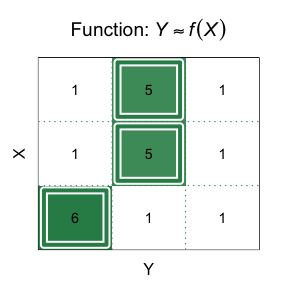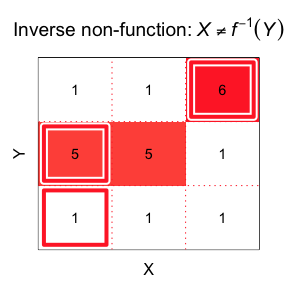#> Conditional entropy picked CORRECT direction X to Y: H(Y|X) = 0.77 < H(X|Y) = 0.88
#> Original FunChisq picked CORRECT direction X to Y: p(X->Y) = 0.027 < p(Y->X) = 0.059
#> Adapted FunChisq picked CORRECT direction X to Y: p(X->Y) = 0.027 < p(Y->X) = 0.059

### Example 2. A function in a landscape table versus its inverse

In this example, conditional entropy failed to detect the correct functional direction.

func <- matrix(c(
1, 1, 6, 1,
1, 1, 6, 1,
1, 6, 1, 1
), nrow=3, byrow=TRUE)
compare.methods(func)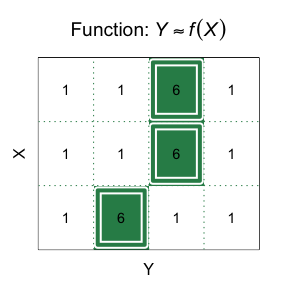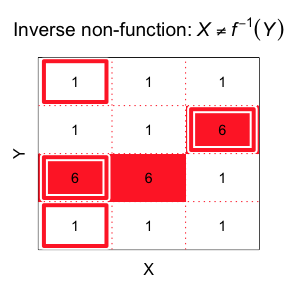#> Conditional entropy picked *WRONG* direction Y to X: H(Y|X) = 1 >= H(X|Y) = 0.9
#> Original FunChisq picked CORRECT direction X to Y: p(X->Y) = 0.022 < p(Y->X) = 0.12
#> Adapted FunChisq picked CORRECT direction X to Y: p(X->Y) = 0.036 < p(Y->X) = 0.12

### Example 3. A function in a portrait table versus its inverse

In this example, the original FunChisq could not detect the correct functional direction.

func <- matrix(c(
1, 5,
1, 6,
4, 1
), nrow=3, byrow=TRUE)
compare.methods(func)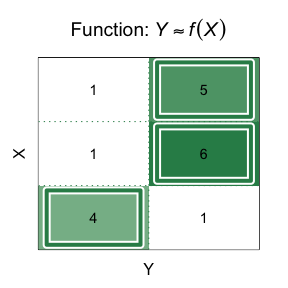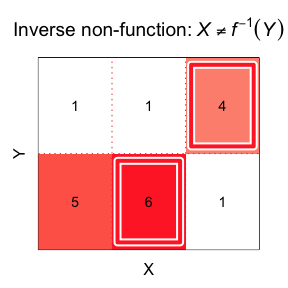#> Conditional entropy picked CORRECT direction X to Y: H(Y|X) = 0.45 < H(X|Y) = 0.9
#> Original FunChisq picked *WRONG* direction Y to X: p(X->Y) = 0.049 >= p(Y->X) = 0.046
#> Adapted FunChisq picked CORRECT direction X to Y: p(X->Y) = 0.049 < p(Y->X) = 0.76

## Detecting functional strength

The $$p$$-value of the AdpFunChisq is also a good indicator of the strength of a functional relationship $$Y=f(X)$$ over an independent relationship where $$X \perp Y$$.

### Example 4. Functional versus independent patterns

In this example, conditional entropy failed to prioritize a functional pattern over an empirically independent pattern which is also a constant function.

# approximately functional pattern
func = matrix(c(
8, 0, 1, 0,
1, 8, 1, 1,
8, 1, 1, 0
), nrow=3, ncol=4, byrow=TRUE)

# empirically independent (constant) pattern
const = matrix(c(
9, 0, 0, 0,
11, 0, 0, 0,
10, 0, 0, 0
), nrow=3, ncol=4, byrow=TRUE)

# calculate the AdpFunChisq P-value of 'func' and 'const' tables, lower the better.

# calculate the Conditional Entropy scores of 'func' and 'const' tables, lower the better.
sample.matrix = Untable(func)
x <- as.numeric(sample.matrix[,1])
y <- as.numeric(sample.matrix[,2])
func_H = condentropy(y, x)

sample.matrix = Untable(const)
x <- as.numeric(sample.matrix[,1])
y <- as.numeric(sample.matrix[,2])
const_H = condentropy(y, x)

# plot table with AdpFunChisq and Conditional Entropy Scores
# functional pattern
plot_table(
func, xlab = 'Y', ylab = 'X', value.cex = 0.9, cex.main=0.9, col="seagreen",
main=paste0("AdpFunChisq P = ", format(func_afc$p.value, digits = 2), "\nConditional Entropy = ", format(func_H, digits = 2))) # constant pattern plot_table( const, xlab = 'Y', ylab = 'X', value.cex = 0.9, cex.main=0.9, col="firebrick1", main=paste0("AdpFunChisq P = ", format(const_afc$p.value, digits = 2),
"\nConditional Entropy = ", format(const_H, digits = 2)))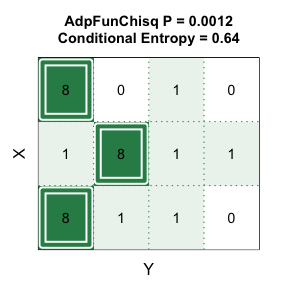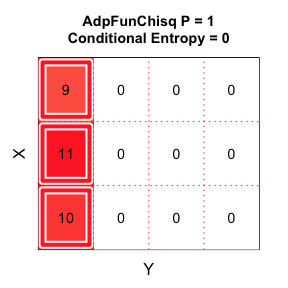Here, AdpFunChisq correctly rated the functional pattern ($$p$$-value=0.001181) stronger than the independent pattern ($$p$$-value=1); while conditional entropy incorrectly rated the former more poorly (0.6423708) than the latter (0).

## Conclusion

In functional inference model free, we recommend the adapted FunChisq test when tables are of different shape or dimension; otherwise, the original FunChisq test is adequate. We generally do not recommend conditional entropy for inferring non-constant function patterns.